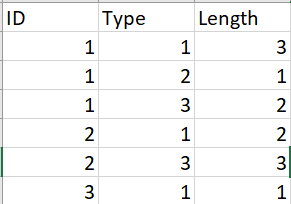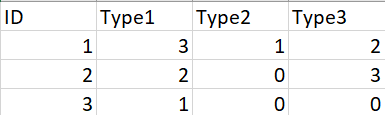## How do I rearrange my columns to rowsmy data looks like this nowI'm wondering if is there a way that I could transfer the 'Length' variable into a corresponding table with x-axis "Type' and y-axis 'ID'?

Thank you!

1 ACCEPTED SOLUTION

Accepted Solutions

## Re: How do I rearrange my columns to rows

You erroneously posted this in the Graphics community, do you got some misleading answers. I moved this to Programming.

Use PROC TRANSPOSE:

``````proc transpose
data=have
out=want (rename=(_name_=type col1=length))
;
by id;
var type:;
run;``````
7 REPLIES 7

## Re: How do I rearrange my columns to rows

Hi:
PROC REPORT could generate the table you show. I am confused over whether you want a report table, a graph image or an transposed dataset?
Cynthia

## Re: How do I rearrange my columns to rows

Hi, I want a transposed dataset. The problem for me now is that I want to merge two datasets, but one of them contains multiple observations under the same ID, like what shows in the first table. Since both type and length are the required variables I want to use in the merged new dataset and they are corresponded, I'm trying to find a way that is able to combine them together. For example, since I have three categories of Type in the first table, I want to make three new variables: 'Type 1', 'Type 2' and 'Type 3'; and under each type, I want to put in the corresponding 'Length' number

## Re: How do I rearrange my columns to rows

It is not clear which is your starting data and which is the one that you want.

If you want to graph anything that first appearance of data is likely much nicer in the long run.

```Proc sgplot data=have;
scatter x=type y=id / group=length;
run;```

For example will create a scatter plot with a different marker/color combination for each value of length. Many values of length can make the graph busy with multiple symbol/colors.

```Proc sgpanel data=have;
panelby length;
scatter x=type y=id;
run;```

The above will create a graph with separate panels for values of length (though lots of values may require some additional options to look nice).

## Re: How do I rearrange my columns to rows

Hi, I want a transposed dataset. The problem for me now is that I want to merge two datasets, but one of them contains multiple observations under the same ID, like what shows in the first table. Since both type and length are the required variables I want to use in the merged new dataset and they are corresponded, I'm trying to find a way that is able to combine them together. For example, since I have three categories of Type in the first table, I want to make three new variables: 'Type 1', 'Type 2' and 'Type 3'; and under each type, I want to put in the corresponding 'Length' number

## Re: How do I rearrange my columns to rows

You erroneously posted this in the Graphics community, do you got some misleading answers. I moved this to Programming.

Use PROC TRANSPOSE:

``````proc transpose
data=have
out=want (rename=(_name_=type col1=length))
;
by id;
var type:;
run;``````

## Re: How do I rearrange my columns to rows

Thank you!Ksharp
Super User

## Re: How do I rearrange my columns to rows

``````/*Simple PROC TRANSPOSE*/
data have;
input id type length;
cards;
1 1 3
1 2 1
1 3 2
2 1 2
2 3 3
3 1 1
;
proc transpose data=have out=temp(drop=_name_) prefix=Type;
by id ;
var length;
id type;
run;
proc stdize data=temp out=want reponly missing=0;
run;``````
Discussion stats
• 7 replies
• 252 views
• 1 like
• 5 in conversation